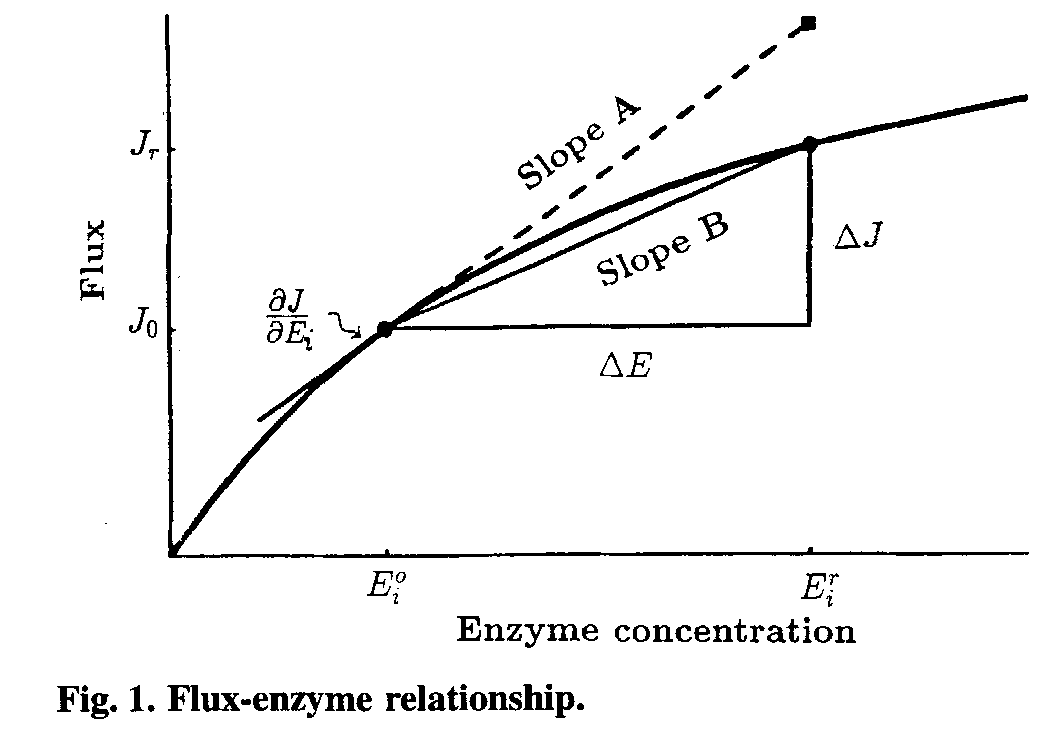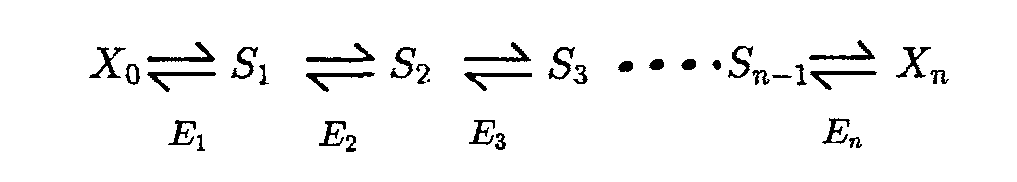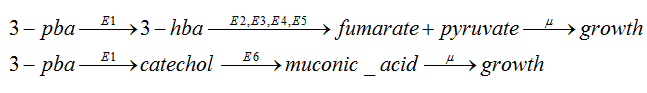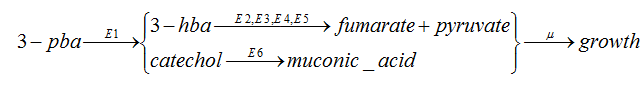# Metabolic control analysis

Metabolic control analysis(MCA) can be used to elucidate the control structure of the metabolic network.Generally,central to MCA are elasticity coefficients and flux control coefficients(FCCs),two groups coefficients which define the properties of the system at steady state.

At first,we’ll introduce the metabolic flux.J is define as the metabolic flux,which represent the metabolic or production rate by every unit mass:

J_i=nu_i/x

Elasticity coefficients (epsilon_(x_j)^i) represent local properties of the metabolic network and are a measure of hoe the individual metabolite concentrations of a metabolic pathway influence the rate of a specific enzymatic step[].

epsilon_(x_j)^i=c_j/nu_i(delnu_i)/(delc_j) (i in{1,2,...,L},jin{1,2,...,K})

FCCs (C_i^{J_k}) on the other hand,represent systematic properties of the network and determine how the overall flux of the pathway is influenced by a given reaction[].As they are defined in the form of relative flux and relative enzyme activity,these coefficients are dimensionless.

C_i^{J_k}=E_i/J_k(dJ_k)/(dE_i) (i,j in{1,2,...,L})

Since the two group of coefficients are related by the connectivity and summation theorems,

 Connectivity theorems: (when the metabolic system involved with K kinds of metabolites and L kinds of fluxes): {sum_(i=j)^L C_i^(J_k)epsilon_(X_j)^i=0 --(i in {1,2,...,L},j in {1,2,...,K})} Summation theorems: sum_(i=1)^L C_i^(J_k)=1 (k in {1,2,...,L})

many people choose to use the Elasticity coefficients to calculate the FCCs,and the elasticity coefficients can be gained when developed a correct dynamic model.But we can not be sure that the dynamic model we built at first can accurately represent the authentic kinetic reaction.Whether the enzymatic reactions are reversible is unknown.So,we chosen another method to calculate the FCCs--the large-perturbation theory.

 Large-perturbation theory:The FCCs are defined as C_i^(J_k)=E_i/(J_k)(dJ_k)/(dE_i), so they are shown in Fig.1 as the slopeA of the tangent.If we want to gain FCCs directly from experiment,very small changes in enzyme activity are needed.We’ll face the problem that small changes may cause large measurement error while large changes will get the slopeB(Fig.1),the wrong result.But the problem can be solved by large-perturbation theory. A Deviation Index, D, is defined, as follow: D_(E_i^r)^(J^r)=((Delta J)/(DeltaE_i))*E_i^r/(J^r),where Delta J=J^r-J^0 ,and DeltaE_i=E^r-E^0, J^r=resulting flux after the enzyme activity has changed by a factor r.Both the control coefficient and the deviation index are dimensionless numbers and, in format, are similar. To make an accurate prediction with large changes ,it is necessary to find the relationship, if any, between the control coefficient and the deviation index. This general problem cannot be solved since it involves the solution of many simultaneous, non-linear, equations, which cannot be achieved algebraically. If, however, we make certain assumptions about the rate equations governing each step in our system the equations are soluble. The assumption is that the rate of a reaction is, effectively, a linear function of the metabolites which affect it. The reaction E+X_i iff EX_i iff E+X_j can be described as the function nu=(nu_(max)^F c_i//K_m^F-nu_(max)^R c_j//K_m^R)/(1+c_i//K_m^F+c_j//K_m^R) .Then it will be simplified to nu=e(c_i-c_j/K) ,when e=c(V_max^F)/(K_m^Fk_s);K=1//((nu_max^R)/(K_m^R)(K_m^F)/(nu_max^F));k_s=1+c_i/(K_m^F)+c_j/(K_m^R) When it comes to a linear pathway as follow:We can get the J in steady state as J=(c_0-c_n//K_(1:n))/(1/E_1+1/(E_2K_1)+1/(E_3K_(1:2))+...+1/(E_nK_(1:n-1))),when define K_(1:n)=prod_(i=1)^n K_i. If we only change the enzyme i activity,then we can get a simplified function that : J=(c_0-c_n//K_(1n))/(1//E_i K_(1:i-1)+C)=((c_0-c_n//K_(1:i-1))K_(1:i-1)E_i)/(1+CK_(1:i-1)E_i) ,where C is a constant. If we keep the and changeless,the function can become J=(E*chi_(1,i))/(1+E*chi_(2,i)). So C_i^J=1/(1+E_i^0*chi_(2,1)). We also know that r=E_i^r/E_i^0, then, we can get J_i^0=(E_i^0*chi_(1,i))/(1+E_i^0*chi_(2,i)), and J_i^r=(r*E_i^r*chi_(1,i))/(1+r*(E_i^r)*chi_(2,i)). Then, the slope is:(Delta J)/(Delta E)=(J^r-J^0)/(E^r-E^0)=chi_(1,i)/((1+r*E_i^0*chi_(2,i))(1+E_i^0*chi_(2,i))), while the E_i^r/J^r=(1+r*E_i^0*chi_(2,i))/chi_(1,i). (Delta J)/(Delta E) E_i^r/(J^r)=1/(1+E_i^0*chi_(2,i))=D_(E_i^r)^(J^r), that is D_(E_i^r)^(J^r)=C_i^J.

In our system,we have the network as follow:As the 3-hba and catechol will be metabolized separately,so we can regard the pathway as two linear pathway:When there is in steady state, nu_1=nu_2=nu_3, that is the kinetic rates are the same as the 3-pba degradation rate.

## Experiment

In the experiment,set the initial rate as nu_0, then change the E_i in the network,getting the nu_r. If we define Delta J=Delta nu=nu_r-nu_0, We'll get C_i^J(i=1,2,3,4,5,6). Comparing C_1^J, C_2^J, C_3^J, C_4^J, C_5^J and C_6^J, find the max of them,which is the key enzyme in the network.If we over express the key enzyme and less express the enzyme which has the FCCs nearly equal 0,we'll achieve the goal in benefit-cost analysis part,that produce less protein while gain more energy and be more efficient.

Reference

1. Angela Cintolesi, J.M.C., 1 Venetia Rigou,1 Kyriacos Zygourakis,1,2 and Ramon Gonzalez1, < Quantitative Analysis of the Fermentative Metabolism of Glycerol in Escherichia coli >. 3 June 2011.
2. KACSER, J.R.S.a.H., Responses of metabolic systems to large changes in enzyme activities and effectors. July 21, 1992.